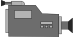# 5D: Writing Equations

This tutorial describes how to write equations from a 5D table.

5D: Writing Equations